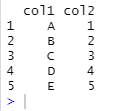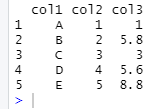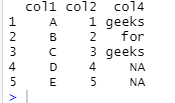Open In App

# Insert list as dataframe column in R

In this article, we will discuss how to add a list as a column to dataframe in R Programming Language.

Creating dataframe for demonstration:

## R

 `df<-``data.frame``(col1 = ``c``(``'A'``, ``'B'``, ``'C'``, ``'D'``, ``'E'``),``               ``col2 = ``c``(1, 2, 3, 4, 5))`` ` `df`

Output:Now to add a list as a column, create a list with required values. Then, use the name of the data frame and the new column separated by \$ and assign this to the list so created.

Syntax: dataframe\$column_name=list

This will assign the list to the column name given and then add it to the dataframe.

## R

 `df <- ``data.frame``(col1 = ``c``(``'A'``, ``'B'``, ``'C'``, ``'D'``, ``'E'``),``                 ``col2 = ``c``(1, 2, 3, 4, 5))`` ` `new_col = ``list``(1.0, 5.8, 3.0, 5.6, 8.8)``df\$col3 <- new_col`` ` `df`

Output:Creating columns in such a way allows adding NA values where no data is required too. Just write NA for places where data is not there and the rest of the process is the same as above.

Example:

## R

 `df <- ``data.frame``(col1 = ``c``(``'A'``, ``'B'``, ``'C'``, ``'D'``, ``'E'``),``                 ``col2 = ``c``(1, 2, 3, 4, 5))`` ` `new_col=``list``(``'geeks'``,``'for'``,``'geeks'``, ``NA``, ``NA``)``df\$col4<-new_col`` ` `df`

Output: